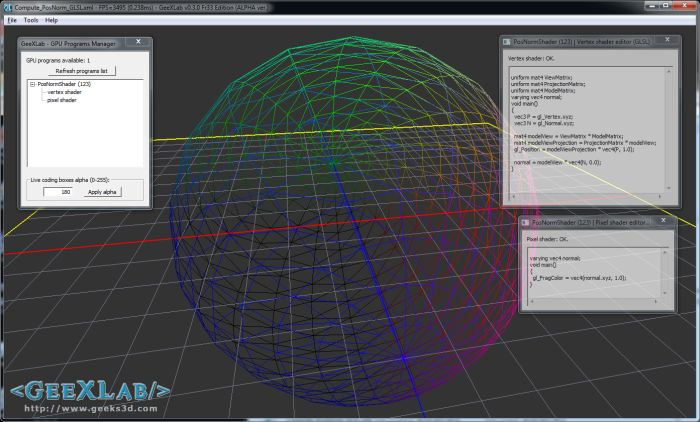# How to Compute the Position and Normal in the Vertex Shader in OpenGL (GLSL) and Direct3D (HLSL)This howto is valid for all versions of OpenGL (2, 3, and 4) as well as all version of Direct3D (9, 10 and 11). I give code snippets in OpenGL 2 and Direct3D 9. OpenGL 3 / 4 or Direct3D 10 / 11 codes can be easily derived from the GL2 / D3D9 ones.

To compute the tranformed position (gl_Position in GLSL, clipping space), you need three matrices: projection, view and model. Projection and view matrices are camera matrices and model matrix is the transformation matrix of the current object being rendered.

The three matrices are passed to the vertex shader as input variables (uniform in GLSL) by the host application.

In the following vertex shaders, P is the XYZ position of the vertex in local space or object space: the position without any transformation. Same thing for N, the vertex normal in local space without any transformation.

OpenGL 2 / GLSL vertex shader:

```uniform mat4 projectionMatrix;
uniform mat4 viewMatrix;
uniform mat4 modelMatrix;

varying vec3 normal;

void main()
{
vec3 P = gl_Vertex.xyz;
vec3 N = gl_Normal.xyz;

mat4 modelView = viewMatrix * modelMatrix;
mat4 modelViewProjection = projectionMatrix * modelView;
gl_Position = modelViewProjection * vec4(P, 1.0);

normal = modelView * vec4(N, 0.0);
}
```

Direct3D 9 / HLSL vertex shader:

```float4x4 projectionMatrix;
float4x4 viewMatrix;
float4x4 modelMatrix;

struct VS_OUTPUT
{
float4 Position : POSITION;
float3 Normal: TEXCOORD0;
};

VS_OUTPUT VertexShaderD3D(float4 P : POSITION, float3 N : NORMAL)
{
VS_OUTPUT o;
float4x4 modelView = mul(modelMatrix, viewMatrix);
float4x4 modelViewProjection = mul(modelView, projectionMatrix);
o.Position = mul(float4(P.xyz, 1.0), modelViewProjection);
o.Normal = mul(N, (float3x3)modelView);
return o;
}
```

Remark: OpenGL matrix convention is column-major order while Direct3D convention is row-major order. That explains why the order of matrices multiplication is not the same in OpenGL and Direct3D.

And if you want to play with the GLSL shader (with live coding), you can find it in GeeXlab code samples pack in the GLSL_ComputePosNorm/ folder of the zip archive. The latest version of GeeXLab is available HERE.Just launch GeeXLab and drag-n-drop the demo file (GLSL_Compute_PosNorm.xml) into GeeXLab, that’s all.

## 8 thoughts on “How to Compute the Position and Normal in the Vertex Shader in OpenGL (GLSL) and Direct3D (HLSL)”

1.Daniel Rakos

Wow, well, my advice is to never do something this:

mat4 modelView = viewMatrix * modelMatrix;
mat4 modelViewProjection = projectionMatrix * modelView;
gl_Position = modelViewProjection * vec4(P, 1.0);

This involves 2 matrix-matrix multiplication and 1 matrix-vector multiplication. This is *very* expensive. If I count it correctly it’s usually a total number of 2*16+4=36 GPU instructions (36 dot products).

Why not do it in the following way?

vec4 modelSpacePos = modelMatrix * vec4(P, 1.0);
vec4 viewSpacePos = viewMatrix * modelSpacePos;
gl_Position = projectionMatrix * viewSpacePos;

This way it is much faster. If I count it correctly now we only need 3*4=12 GPU instructions (12 dot products).

2.JeGX Post Author

Thanks Daniel! Will test it on a massive mesh to see the impact of your nice optimization.

3.Daniel Rakos

HiroshimaCC:
Yes, in fact the normal should be multiplied by the inverse-transpose of the modelview matrix, but if you check the math, the inverse-transpose of an orthogonal matrix (which is usually true for modelview matrices) is actually the matrix itself.

4.mg

– just to follow up on what Daniel, the matrix is usually orthonormal if you are NOT using non-uniform scaling, or skew-matrices.

Also, an alternative to what Daniel suggested is to multiply the matrices app-side, and pass in the modelMatrix, modelViewMatrix, and modelViewProjectionMatrix.

5.HiroshimaCC

Yes, IF it’s orthogonal, which happens USUALLY, but as I said, not ALWAYS. Trying to found out the bug of why your normals are off become tricky espacially if your are new at programming shader (it was my case and I struggled to find out why at the time).

So mentionning it is at least interesting, and OpenGL provide glNormalMatrix, so why not using it ?

6.JeGX Post Author

gl_NormalMatrix is deprecated in OpenGL 3/4 core profile as well as gl_ModelViewMatrix and gl_ProjectionMatrix.

7.Kurious

There is a bug in the GLSL code.
varying vec3 normal;

should be

varying vec4 normal; // as its writing to vec4 value